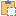#### BoundingBoxXYZ Class

A three-dimensional rectangular box at an arbitrary location and orientation within the Revit model.

Namespace: Autodesk.Revit.DB
Assembly: RevitAPI (in RevitAPI.dll) Version: 21.0.0.0 (21.1.1.109)

# Syntax

C#
``public class BoundingBoxXYZ : APIObject``
Visual Basic
``````Public Class BoundingBoxXYZ _
Inherits APIObject``````
Visual C++
``public ref class BoundingBoxXYZ : public APIObject``

# Remarks

BoundingBoxXYZ objects are used in Revit in several places related to views (for example, the section box of a 3D view or the definition of a section or detail view). BoundingBoxXYZ objects can also be obtained from elements representing the boundary of the element in a given view.

The extents of the box are determined by three orthogonal planes extended through the minimum ( Min ) and maximum ( Max ) points, but the coordinates of these points and the orientation of the planes in relation to the coordinates of the source model is determined by the box Transform ( Transform ).

This class also has the ability to detect and mark certain extents as disabled. Note that in the current Revit API uses of this class it is not expected that Revit will give objects with disabled extents, and disabled extents in objects sent to Revit will likely be ignored.

# ExamplesCopy C#
``````public void GetBoundingBoxXYZInfo(View3D view3D)
{
string message = "BoundingBoxXYZ : ";
message += "\nView name : " + view3D.Name;
BoundingBoxXYZ boundingBox = view3D.GetSectionBox();
// The section box of the 3D view can cut the model.
if (view3D.IsSectionBoxActive)
{
BoundingBoxXYZ sectionBox = view3D.GetSectionBox();

// Note that the section box can be rotated and transformed.
// So the min/max corners coordinates relative to the model must be computed via the transform.
Transform trf = sectionBox.Transform;

XYZ max = sectionBox.Max; //Maximum coordinates (upper-right-front corner of the box before transform is applied).
XYZ min = sectionBox.Min; //Minimum coordinates (lower-left-rear corner of the box before transform is applied).

// Transform the min and max to model coordinates
XYZ maxInModelCoords = trf.OfPoint(max);
XYZ minInModelCoords = trf.OfPoint(min);

message += "\nView has an active section box: ";
message += "\n'Maximum' coordinates: " + maxInModelCoords;
message += "\n'Minimum' coordinates: " + minInModelCoords;
}
}``````Copy VB.NET
``````Public Sub GetBoundingBoxXYZInfo(view3D As View3D)
Dim message As String = "BoundingBoxXYZ : "
message += vbLf & "View name : " + view3D.Name
Dim boundingBox As BoundingBoxXYZ = view3D.GetSectionBox()
' The section box of the 3D view can cut the model.
If view3D.IsSectionBoxActive Then
Dim sectionBox As BoundingBoxXYZ = view3D.GetSectionBox()

' Note that the section box can be rotated and transformed.
' So the min/max corners coordinates relative to the model must be computed via the transform.
Dim trf As Transform = sectionBox.Transform

Dim max As XYZ = sectionBox.Max
'Maximum coordinates (upper-right-front corner of the box before transform is applied).
Dim min As XYZ = sectionBox.Min
'Minimum coordinates (lower-left-rear corner of the box before transform is applied).
' Transform the min and max to model coordinates
Dim maxInModelCoords As XYZ = trf.OfPoint(max)
Dim minInModelCoords As XYZ = trf.OfPoint(min)

message += vbLf & "View has an active section box: "
message += vbLf & "Maximum' coordinates: " + maxInModelCoords.ToString()
message += vbLf & "Minimum' coordinates: " + minInModelCoords.ToString()
End If